Hydrol. Earth Syst. Sci., 24, 4001–4024, 2020
https://doi.org/10.5194/hess-24-4001-2020
Hydrol. Earth Syst. Sci., 24, 4001–4024, 2020
https://doi.org/10.5194/hess-24-4001-2020

Research article 18 Aug 2020

Research article | 18 Aug 2020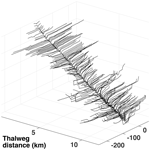# A new form of the Saint-Venant equations for variable topography

A new form of the Saint-Venant equations for variable topography
Cheng-Wei Yu1, Ben R. Hodges1, and Frank Liu2 Cheng-Wei Yu et al.
• 1National Center for Infrastructure Modeling and Management, The University of Texas at Austin, Austin, Texas, USA
• 2Oak Ridge National Laboratory, Oak Ridge, Tennessee, USA

Correspondence: Ben R. Hodges (hodges@utexas.edu)

Abstract

The solution stability of river models using the one-dimensional (1D) Saint-Venant equations can be easily undermined when source terms in the discrete equations do not satisfy the Lipschitz smoothness condition for partial differential equations. Although instability issues have been previously noted, they are typically treated as model implementation issues rather than as underlying problems associated with the form of the governing equations. This study proposes a new reference slope form of the Saint-Venant equations to ensure smooth slope source terms and eliminate one source of potential numerical oscillations. It is shown that a simple algebraic transformation of channel geometry provides a smooth reference slope while preserving the correct cross-section flow area and the total Piezometric pressure gradient that drives the flow. The reference slope method ensures the slope source term in the governing equations is Lipschitz continuous while maintaining all the underlying complexity of the real-world geometry. The validity of the mathematical concept is demonstrated with the open-source Simulation Program for River Networks (SPRNT) model in a series of artificial test cases and a simulation of a small urban creek. Validation comparisons are made with analytical solutions and the Hydrologic Engineering Center's River Analysis System (HEC-RAS) model. The new method reduces numerical oscillations and instabilities without requiring ad hoc smoothing algorithms.

Share
1 Introduction

The Saint-Venant equations (SVEs) for one-dimensional (1D) river modeling are typically presented with pressure forcing terms of either (i) gradients of the water surface elevation or (ii) thalweg bottom slope combined with gradients of the water depth. In this study, we demonstrate a new form using a reference slope (SR) and its associated depth (ha), which are shown to be algebraically identical to the two standard forms of the SVEs. The new forms provide greater flexibility in addressing numerical convergence issues associated with modeling discontinuous bottom slopes. A key point of this paper is that precise representation of the thalweg bottom slope (S0) and hydrostatic pressure gradients ($\partial {h}_{\mathrm{0}}/\partial x$) is not necessary to correctly represent variable topography. Indeed, the splitting point for representing the forcing Piezometric pressure gradient as a body force (defined by a slope) and a residual head gradient term is free choice in a simple algebraic substitution. Different choices for the splitting provide different body force directions and lead to different forms of the SVEs – all of which are valid representations of variable topography and do not constitute a smoothing of topography. We will show that it is possible to use a smooth slope (body force) term in the SVEs without actually smoothing the topography. Herein, this smooth slope term will be designated as the reference slope, SR, to distinguish it from the traditional thalweg bottom slope, S0.

The two common differential forms of the SVEs are

$\begin{array}{}\text{(1)}& \frac{\partial Q}{\partial t}+\frac{\partial }{\partial x}\left(\frac{{Q}^{\mathrm{2}}}{A}\right)=-gA{S}_{\mathrm{f}}-gA\frac{\partial \mathit{\eta }}{\partial x}\end{array}$

and

$\begin{array}{}\text{(2)}& \frac{\partial Q}{\partial t}+\frac{\partial }{\partial x}\left(\frac{{Q}^{\mathrm{2}}}{A}\right)=-gA{S}_{\mathrm{f}}+gA{S}_{\mathrm{0}}-gA\frac{\partial {h}_{\mathrm{0}}}{\partial x},\end{array}$

where Q is the flow rate, A is the cross-section area, η is the water surface elevation, h0 is the thalweg depth, S0 is the thalweg bottom slope, and Sf is the friction slope that represents the local energy gradient. Equation (1) can be envisioned as using the Piezometric head gradient to force the flow, as shown in Fig. 1a. In contrast, Eq. (2) can be envisioned as splitting the Piezometric gradient into a body force in the bottom slope direction and a hydrostatic head gradient, as shown in Fig. 1b. Note that both equations are valid for variable topography despite only the second equation explicitly representing the bottom slope and thalweg depth hydrostatic pressure gradients.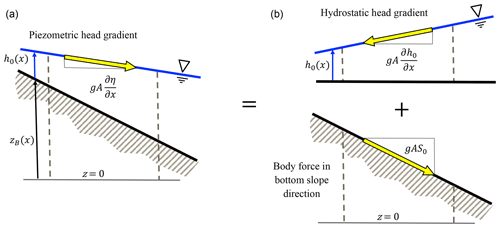Figure 1The η form of the SVEs (a) has a driving Piezometric head gradient which is equivalent (b) to the sum of the hydrostatic head gradient and a body force aligned with S0. The effect of varying geometry is handled in A in both forms.

The two SVE forms of Eqs. (1) and (2) are algebraically identical using the identity

$\begin{array}{}\text{(3)}& \frac{\partial \mathit{\eta }}{\partial x}\equiv \frac{\partial {h}_{\mathrm{0}}}{\partial x}-{S}_{\mathrm{0}}.\end{array}$

Heuristically, we can propose a more general identity of

$\begin{array}{}\text{(4)}& \frac{\partial \mathit{\eta }}{\partial x}\equiv \frac{\partial {h}_{\mathrm{a}}}{\partial x}-{S}_{\mathrm{R}},\end{array}$

where SR is an arbitrary reference slope and ha is an associated depth that will be defined in Sect. 3.2 below. For an introductory exposition, $\partial {h}_{\mathrm{a}}/\partial x$ is merely the residual implied for a given $\partial \mathit{\eta }/\partial x$ and arbitrary SR. Applying Eq. (4) to (1) provides the following:

$\begin{array}{}\text{(5)}& \frac{\partial Q}{\partial t}+\frac{\partial }{\partial x}\left(\frac{{Q}^{\mathrm{2}}}{A}\right)=-gA{S}_{\mathrm{f}}+gA{S}_{\mathrm{R}}-gA\frac{\partial {h}_{\mathrm{a}}}{\partial x},\end{array}$

where the terms gASR and $gA\frac{\partial {h}_{\mathrm{a}}}{\partial x}$ are algebraically equivalent to those in Eqs. (1) and (2). Clearly, if we let SR equal S0, then ha equals h0 and we recover Eq. (2). Furthermore, if we let SR equal 0, then ha equals η and we recover Eq. (1). The equations are identical with these substitutions, so it follows that using a reference slope of zero (SR=0) must exactly represent the same topographic variability as using a reference slope that mimics the topographic slope (SR=S0) as long as ha is correctly defined consistent with Eq. (4). That is to say that from simple algebra the use of the real S0 in the SVEs is not required to capture effects of topographic variability as long as the depth gradient term is correctly redefined as something other than the thalweg depth gradient and the cross-section flow areas are correctly computed.

From the arguments above, the effects of varying bottom topography are captured by SR=0 and ha=η, which implies we are also free to introduce any other (preferably smooth) SR into Eq. (5) without altering the underlying representation of variable topography. An example is illustrated in Fig. 2. As the splitting defined in Eq. (4) makes Eq. (5) algebraically identical to Eqs. (1) and (2), the introduction of a smooth SR does not reflect “smoothing” of the topography. It merely reflects a decision on whether effects of non-smoothness will reside solely in solution variables A and ha or will also be forced as a non-smooth source term in S0.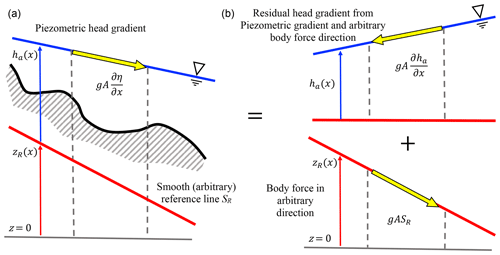Figure 2Comparison of Piezometric forcing terms for varying topography. (a) Eq. (1) with the arbitrary SR line provided for reference. (b) The equivalent split form of forcing with Eq. (5) using the identity of Eq. (4). The physical bottom topography, shown only in (a) for clarity, only plays a role through the cross-section area (A) which feeds back into the solution of variability in ha in both forms of the equation.

We would like to use an a priori smooth SR in a computational model rather than the actual thalweg S0 because of what happens to S0(x) and $\partial {h}_{\mathrm{0}}\left(x\right)/\partial x$ for topography varying sharply over short distances, as illustrated in Fig. 3. From a physics perspective, using S0 to split the Piezometric head is an intuitive way to describe the local interplay of pressure with the bottom slope. Furthermore, S0 has the advantage of being readily reduced to a kinematic wave equation where Sf=S0, which has some advantage in multipurpose codes. However, from a numerical modeling perspective, using S0 has a significant limitation based on its smoothness. If the water surface is smooth, then non-smooth S0(x) requires the numerical solver to produce a compensating non-smooth h0(x), i.e., requiring a well-balanced scheme (see Sect. 2). If we can discard our (wrong) intuition that the S0 form must somehow better represent sharply variable topography – i.e., recognizing the algebraic equivalence of Eq. (5) with Eqs. (1) and (2) – it follows that the splitting of the Piezometric head to include a body force that is everywhere exactly aligned with a sharply varying S0 is (from a numerical perspective) merely creating unnecessary complexity in the governing equation source term that requires compensating complexity in the solution algorithm. In contrast, by requiring SR to be smooth, we can ensure the ha solution is also smooth for a smoothly varying free surface.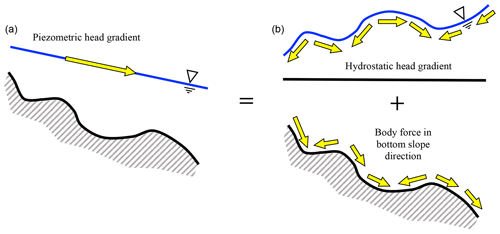Figure 3Comparison of Piezometric forcing terms with sharply varying topography for Eqs. (1) and (2). (a) The Piezometric head gradient for Eq. (1). (b) The equivalent split form of forcing from Eq. (2) using the identity of Eq. (3). The variability of the bottom of (a) will be reflected in the cross-section area, A(x), that feeds back into the solution of η(x). In contrast, (b) attempts to directly represent topographic variability as both a driving term in S0(x) and a solution response term, h0(x)

The use of SR rather than S0 in the governing equations can perhaps be better understood if we think of the slope in Eq. (4) as representing simply a portion of the overall Piezometric pressure gradient that can be extracted from $\partial \mathit{\eta }/\partial x$ and treated as a body force that varies gradually along the channel. Hence, in Fig. 3 we are not interested in separating out the details of the sharply varying slope changes in the local topography but instead prefer a body force term that aligns with the mean slope over some larger spatial scale, e.g., as in Fig. 2.

In this paper, we examine the effect of variable cross-section geometry on numerical solutions of the SVEs and propose a new reference slope approach that can be inferred from the above arguments. The use of a reference slope as a body force direction to split the Piezometric head gradient term ensures (i) the slope used in the discrete source term is smooth, (ii) the variable geometry is correctly retained, (iii) the fundamental governing equations are preserved, and (iv) an SVE numerical algorithm developed using S0 is essentially unchanged. We further demonstrate that bottom slope discontinuities are a cause of problems in finite-difference forms of 1D Saint-Venant equations with subcritical flow. Of course, this idea will not be a surprise to many modelers who routinely remove troublesome cross sections or smooth their topography; however, the concept does not appear to have been conclusively demonstrated in the literature. More importantly, we show that the problem is inherent in the traditional formulation of the governing equations using the thalweg bottom slope, S0, which is usually computed as the slope between the lowest points in two adjacent river cross sections. Problems associated with slope discontinuities can be fixed within the governing equations by the careful selection of smoothly changing reference elevations along the channel, zR(x), which results in smooth reference slopes, SR(x), and the redefinition of the thalweg depth (h0) as a depth associated (ha) with the reference elevation. Note that ha is not necessarily any characteristic depth of the flow and is only indirectly related to the hydrostatic pressure.

The approach proposed herein can be implemented within any Saint-Venant model as it is entirely independent of the solution algorithm; however, implementation does require rewriting code for the relationships between cross-section area, wetted perimeter, and the depth variable of the solution. Note that the new approach includes a redefinition of the depth variable (traditionally the maximum depth, h0) as the depth associated (ha) with the reference elevation, zR.

2 Background

One-dimensional (1D) hydrodynamic models using the Saint-Venant equations (SVEs) are widely employed for studying both natural streams and man-made channels (e.g., Martinez-Aranda et al.2019; Sanders2001). It is widely recognized that numerical solutions of the SVEs are prone to spurious oscillations in the free-surface elevation unless particular care is taken in the numerical formulation and/or the problem definition (e.g., Nujic1995; Tseng2004). Numerous techniques and special numerical schemes have been previously designed to overcome unwanted numerical oscillations caused by discontinuous geometries and boundary conditions (e.g., Zhou et al.2001; Liang and Marche2009). These approaches typically rely on the concept of a well-balanced discrete form as discussed in a comprehensive review by and further elaborated by .

Although well-balanced schemes are relatively robust in handling discontinuous boundary conditions, they have not been extensively applied in water resource models to simulate the regional-to-continental-scale river networks or stormwater systems for megacities. The rapidly varying and discontinuous S0 in natural systems can significantly increase the difficulty and computational burden of obtaining a well-balanced method . Hence, when a large-scale open-channel model develops oscillations and/or instabilities, practitioners may resort to the traditional approach of removing cross sections or smoothing bathymetry to mitigate oscillatory or unstable solution behavior . Such ad hoc efforts can be effective as they address a major cause of such oscillations and instabilities (discontinuous topography), but they inherently reduce the fidelity of the simulation.

Oscillations and instabilities can be induced in any numerical solution of a boundary initial value problem by the inclusion of non-smooth source terms; i.e., if we consider an advection equation of the form

$\begin{array}{}\text{(6)}& \frac{\partial Q}{\partial t}+\frac{\partial }{\partial x}\left(\frac{{Q}^{\mathrm{2}}}{A}\right)=\mathit{\sigma },\end{array}$

where σ is a nonhomogeneous source term, a fundamental theorem for differential equations provides that a unique solution cannot be guaranteed to exist unless the source term is Lipschitz continuous (e.g., Iserles1996). That numerical instabilities are often caused by non-smooth source terms is not a new observation. A wide variety of numerical schemes have been developed to address this issue, including, e.g., extensive work on wetting/drying , positivity-preserving methods for coupled models , and implicit schemes that address stiffness of the nonlinear friction term . The literature in this area is vast – particularly if both 1D and 2D models are considered. For the present purposes, we focus on only one part of the source term, S0, whose non-smoothness has previously been treated as a problem to be handled rather than as a problem that can be directly eliminated in the governing equations. Existing well-balanced schemes (see reviews noted above) seek to compensate for non-smoothness of all parts of the source term in the structure of the numerical discretization. Arguably, if the slope term is guaranteed smooth, then a well-balanced scheme should be simpler to create. In general, when the thalweg bottom slope (S0) appears as a source term in the SVEs, it should be a priori Lipschitz smooth or oscillations and instabilities should be expected. For natural systems, S0(x) is typically defined using the maximum channel depth at each surveyed cross section, which is rarely a smooth function (unless the distance between cross sections is large compared to bottom elevation variability). When cross sections are surveyed at short distances, S0 will tend to have significant variability. It follows that the use of S0 has the undesirable property that smaller Δx (i.e., resolving a river with more detailed survey data) will increase the non-smoothness in this source term of the momentum equation, resulting in a model that is unlikely to converge under a grid refinement test. It is not surprising that S0 smoothness, when it occurs in a model of a natural river channel, is typically the result of relatively long separations (Δx) between cross-section surveys that ensures that the discrete d2z0∕dx2 is small. Thus, removing cross sections can be an effective mitigation technique because it increases Δx and effectively smooths S0. In general, models discretized with higher-resolution river surveys (smaller Δx) will have greater non-smoothness in S0 and develop more oscillation and instability issues. In essence, our models get worse as our boundary condition data get better.

The problems associated with S0 can be understood by considering the identity in Eq. (3) for a channel with subcritical flow in which the free-surface curvature is expected to be negligible, i.e., ${\partial }^{\mathrm{2}}\mathit{\eta }/\partial {x}^{\mathrm{2}}\sim \mathit{ϵ}$, where $\mathit{ϵ}\ll \text{d}{S}_{\mathrm{0}}/\text{d}x,{\partial }^{\mathrm{2}}\mathit{\eta }/{\partial }^{\mathrm{2}}x$. Taking the along-channel gradient of Eq. (3) implies that

$\begin{array}{}\text{(7)}& \frac{{\partial }^{\mathrm{2}}{h}_{\mathrm{0}}}{\partial {x}^{\mathrm{2}}}=\frac{\text{d}{S}_{\mathrm{0}}}{\text{d}x}-\mathit{ϵ}.\end{array}$

Thus, forcing with a non-smooth dS0(x)∕dx will require non-negligible curvature of the response variable, h0(x), whose gradient is also a forcing function of the nonlinear equation. Feedback can easily build and cause successive overshoot/undershoot effects, producing oscillations and non-convergence in a nonlinear solver. In contrast, Eq. (4) can be invoked with dSR∕dx guaranteed to be small, which implies that ${\partial }^{\mathrm{2}}{h}_{\mathrm{a}}/\partial {x}^{\mathrm{2}}$ will also be small even when $\partial A/\partial x$ is non-smooth. As a practical matter, any S0 with a discontinuous discrete first derivative (i.e., discontinuities in the second derivative of the thalweg elevation, d2z0∕dx2) will be Lipschitz discontinuous and should not be directly discretized in an SVE solution with Eq. (2). Although approximate numerical solutions of equations with non-smooth S0(x) can sometimes be attained for models with sufficient damping, such solutions are questionable as they do not have rigorous mathematical foundations.

Arguably, non-smoothness in S0 can be handled in one of four ways: (i) smoothing the geometry – hence solving for flows that do not match the real system; (ii) applying ad hoc smoothing within the flow solution – i.e., adjusting the physics to remove numerical instabilities; (iii) adjusting the numerical discretization scheme to compensate for non-smoothness – e.g., the well-balanced concept; or (iv) adjusting the governing equations to ensure that any slope in the source term is smooth without modifying the solution physics, the channel geometry, or the numerical discretization scheme. It should be obvious that Eq. (1) is the extreme example of the last approach – replacing S0 and $\partial {h}_{\mathrm{0}}/\partial x$ with $\partial \mathit{\eta }/\partial x$ ensures that S0 does not occur in the governing equations and cannot destabilize the solution. To our knowledge, the last approach (as used herein and illustrated in Fig. 2) has not been previously proposed or analyzed in the literature. Nevertheless, as shown below, it provides a simple method that can be readily adapted into existing hydrodynamic models.

For brevity, we will limit our focus herein to subcritical flows – backwater tends to smooth the effects of slope discontinuities and thus we expect smooth solutions for flow rate and free-surface elevation despite non-smooth geometry. Nevertheless, common SVE solvers can exhibit oscillatory, non-convergent behavior even in simple subcritical flows when geometry is not smooth. In the following, it will be obvious that the mathematical theory applies directly to supercritical and transcritical flows as well, but evaluating model performance under the breadth of possible transcritical conditions (including non-smooth jumps) necessarily requires more analyses than is practical in a single paper.

3 Methods

## 3.1 SPRNT

The Simulation Program for River Networks (SPRNT) code for unsteady SVE river networks is used and modified herein. The baseline for this code models momentum using Eq. (2), which is coupled to the solution of continuity,

$\begin{array}{}\text{(8)}& \frac{\partial A}{\partial t}+\frac{\partial Q}{\partial x}={q}_{\mathrm{\ell }},\end{array}$

where q is a lateral inflow per unit length. Note that significantly non-smooth q(x,t) can provide another source of numerical oscillations and instability . As the main focus of this study is the slope source term in the momentum equation, the lateral inflows and their effects are neglected by setting q=0 everywhere.

In the SPRNT momentum equation, the friction slope is represented using the Chezy–Manning form as

$\begin{array}{}\text{(9)}& {S}_{\mathrm{f}}=\frac{{n}^{\mathrm{2}}{P}_{\mathrm{w}}^{\mathrm{4}/\mathrm{3}}}{{A}^{\mathrm{10}/\mathrm{3}}}{Q}^{\mathrm{2}},\end{array}$

where Pw is the wetted perimeter of a cross section and n is the Gauckler–Manning–Strickler roughness. Although, there are other methods for treating frictional losses (e.g., Decoene et al.2009; Burguete et al.2007), the Chezy–Manning form remains popular due to its simplicity.

The baseline model uses the thalweg elevation (z0), the thalweg depth (h0), and the thalweg bottom slope (S0) as

$\begin{array}{}\text{(10)}& {h}_{\mathrm{0}}\equiv \mathit{\eta }-{z}_{\mathrm{0}}\end{array}$

and

$\begin{array}{}\text{(11)}& {S}_{\mathrm{0}}\equiv -\frac{\partial {z}_{\mathrm{0}}}{\partial x}.\end{array}$

SPRNT is an open-source, 1D hydrodynamic solver using the fully implicit Preissmann numerical scheme with the Newton–Raphson iteration and computational acceleration techniques developed from very large-scale integration (VLSI) semiconductor design. Details on the baseline SPRNT model and its application to large river networks are provided in and .

## 3.2 Reference slope method

We introduce a new reference slope (RS) method through a transformation and redefinition of geometry in the Saint-Venant equations, as discussed in Sect. 1. In place of the conventional h0 and z0, we define a reference elevation (zR) and its associated depth (ha) as shown in Fig. 4. These provide a relationship with the free-surface elevation (η) defined as

$\begin{array}{}\text{(12)}& {h}_{\mathrm{a}}\equiv \mathit{\eta }-{z}_{\mathrm{R}}.\end{array}$

Note that zR is arbitrary, so ha may be either greater than or less than the thalweg depth, h0, at a given location. As shown in Fig. 4, it is convenient to define the reference height, hR, in relation to zR and the true bottom elevation, z0, by

$\begin{array}{}\text{(13)}& {h}_{\mathrm{R}}\equiv {z}_{\mathrm{0}}-{z}_{\mathrm{R}}.\end{array}$

Thus, the conventional h0 and z0 are recovered with

$\begin{array}{}\text{(14)}& {h}_{\mathrm{0}}={h}_{\mathrm{a}}-{h}_{\mathrm{R}}\end{array}$

and

$\begin{array}{}\text{(15)}& {z}_{\mathrm{0}}={z}_{\mathrm{R}}+{h}_{\mathrm{R}}.\end{array}$

We define the reference slope (SR) as the downstream slope of zR:

$\begin{array}{}\text{(16)}& {S}_{\mathrm{R}}\equiv -\frac{\partial {z}_{\mathrm{R}}}{\partial x}.\end{array}$

Using Eqs. (12) and (16) in Eq. (2) provides Eq. (5), which is more conveniently written as

$\begin{array}{}\text{(17)}& \frac{\partial Q}{\partial t}+\frac{\partial }{\partial x}\left(\frac{{Q}^{\mathrm{2}}}{A}\right)=-gA\frac{\partial {h}_{\mathrm{a}}}{\partial x}+gA\left({S}_{\mathrm{R}}-{S}_{\mathrm{f}}\right).\end{array}$

The above is identical to Eq. (2) with the simple substitution of ha and SR for h0 and S0. In this formulation, the definition of zR(x) is arbitrary, so we can a priori require a definition such that SR(x) is smooth. A trivial choice that is guaranteed smooth is zR(x)=constant, which returns SR(x)=0 and the Saint-Venant equations in the form of Eq. (1). However, this form with $\partial \mathit{\eta }/\partial x$ for the entire pressure term is known to cause numerical stiffness issues for large ranges in η, e.g., the elevation change in a river from its mountain source to a coastal plain . Using the conventional S0 in Eq. (2) reduces this problem as the range of h0 is inherently confined to the local water depths rather than the underlying topography. In the RS method, the range of ha is tied to the range of water depths and the selection of zR; thus, for present purposes, we are interested in nontrivial definitions of zR that (i) are close to z0 to maintain a small range of ha values and (ii) provide smooth SR(x). An arbitrary zR that is far from z0 or non-smooth is of little interest as it holds no theoretical or practical advantage over the Eq. (1) approach implied by SR(x)=0.

If SR(x) is required to be smooth, then the source term of the equation can be guaranteed smooth as long as Sf(x) is smooth, which is typically true as long as the solution, Q(x), is smooth. Note that in extreme cases of geometric discontinuity the combined values of n, Pw, and A in Eq. (9) can cause a non-Lipschitz friction term; thus, the RS method cannot guarantee that the entire source term is smooth. Numerical solution methods are usually robust against discontinuities in n and Pw as they are coefficients of the solution variables {A,Q}. More subtle problems might arise due to discontinuities developed in the ${Q}^{\mathrm{2}}{A}^{-\mathrm{10}/\mathrm{3}}$ ratio in Eq. (9); countering incipient instabilities from this term requires other numerical strategies (e.g., Xia and Liang2018).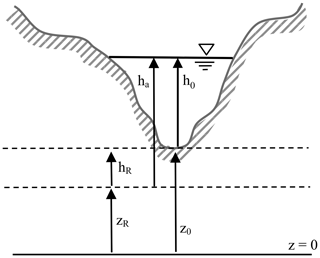Figure 4Relationships of ha, hR, zR, and z0 for an arbitrary cross section. Note that zR>z0 is also allowed, which results in ha<h0 and a negative value for hR. Furthermore, if zR is greater than z0+h0, then ha is negative to retain algebraic consistency. Modified from Liu (2014) and used with permission.

A critical change required by the introduction of ha is that the conventional geometric auxiliary relationships of A=f(h0) and Pw=f(h0) must be transformed into A=f(ha) and Pw=f(ha). That is, once we change the depth in our equation from h0 to ha, we must re-index the geometry. In general, for known functions A(h0) and Pw(h0) this is a trivial transformation:

$\begin{array}{}\text{(18)}& A=A\left({h}_{\mathrm{a}}-{h}_{\mathrm{R}}\right)\end{array}$

and

$\begin{array}{}\text{(19)}& {P}_{\mathrm{w}}={P}_{\mathrm{w}}\left({h}_{\mathrm{a}}-{h}_{\mathrm{R}}\right).\end{array}$

However, the implementation in an existing code is not necessarily as simple as the above equations suggest. For example, in Fig. 4, the condition A=0 occurs when ha=hR, i.e., a nonzero value as compared to h0=0 with conventional geometry. Unfortunately, model developers typically have ad hoc wetting/drying treatments that are introduced as h0→0 or for h0<0. Such treatments need to be modified to be deployed as hahR, which introduces the added complication that hR is negative when zR>z0. Note that the new geometry does not require altering the wetting/drying algorithm itself or, for that matter, any other solution algorithm – only the actual geometry definitions require alteration. These relatively straightforward changes can be contrasted with the effort needed to provide a well-balanced numerical discretization scheme for the conventional S0 representation of geometry (e.g., Kesserwani2013).

The modification of the SPRNT code to implement the above RS method will be known as SPRNT-RS. The SPRNT and SPRNT-RS source codes are available in an open-source repository (Liu2014). Note that the solution algorithm for SPRNT-RS is identical to that of SPRNT; the only code changes are for the new geometry definitions for ha and SR that replace h0 and S0 in the original algorithm. This simple geometry replacement strategy is effective because Eq. (17) is identical to Eq. (2) except for the change in nomenclature to ha and SR.

## 3.3 Generating a smooth SR(x)

The zR(x) and hence SR(x) are arbitrary choices in the RS method but should be generated for the smoothness of SR(x) along the reach. In synthetic reach test cases and analytical test cases (described below), the channels are a priori either specified with a uniform SR or produced by a different order of splines. For our urban creek test case, the zR is generated with an approximating cubic B-spline (de Boor2001) based on thalweg, z0(x), elevations. There are a variety of possible ways to generate smooth SR(x), but applying approximating cubic B-splines to the z0(x) is convenient because the slope is guaranteed to be locally smooth as long as the knot spacing in the B-spline is everywhere larger than the spacing between cross sections. It should be emphasized that an exact spline fitting of all the thalweg data (i.e., knots at all the cross sections) will be smooth at scales finer than the cross-section spacing but non-smooth at the model's discretization scale. That is to say that the exact cubic spline fitting of z0(x) does not reduce discontinuities at the discretization scale – only an approximate fitting associated with coarser scales than the cross-section spacing will be effective. It follows that there is some (limited) choice in the selection of the subset of z0(x) used as the spline knots, with different sets producing slightly different {zR(x),hR(x)} over the domain. Each set is algebraically identical to the underling geometry, so the generated solutions should be identical within the machine truncation error as long as the zR(x) are sufficiently smooth. Implications of the method chosen for generating zR(x) are discussed in Sect. 5.3. Further details and test cases are provided in .

## 3.4 HEC-RAS for model validation

The baseline SPRNT has been previously shown to have excellent agreement with the Hydrologic Engineering Center's River Analysis System (version 5.0.7) – known as HEC-RAS. showed SPRNT simulations agreed with HEC-RAS with ≤3 % difference in water depth solution when using both prismatic cross sections and nonuniform channels. Thus, HEC-RAS provides a reasonable model for testing and validating SPRINT-RS. We would have preferred to use a single model with and without the RS method for such model–model comparisons; however, HEC-RAS is a closed-source proprietary model, so we could not directly implement and test the RS method in that code. Conversely, as expected by the discussions in Sect. 2, the baseline SPRNT model is oscillatory and non-convergent on the highly discontinuous geometry of our test cases due to its use of the S0 approach, so it cannot be directly used for before and after comparisons of RS. Thus, simulations using SPRNT-RS are compared to HEC-RAS simulations for validation and insight.

HEC-RAS provides a convenient validation model for three reasons. Firstly, it is a widely accepted engineering model for river-reach simulations, . Secondly, it has been used as a validation model in numerous prior studies . Finally, unsteady HEC-RAS employs $\partial \mathit{\eta }/\partial x$ as the piezometric gradient rather than using $\partial {h}_{\mathrm{0}}/\partial x$ and S0, which is one of the reasons it is relatively robust for non-smooth geometry such as that used herein.

The performance of the RS method is demonstrated below through (i) a comparison to six analytical test cases from with Lipschitz continuous geometry, various prismatic shapes, and different formulations of SR, (ii) seven synthetic test cases using Lipschitz discontinuous geometry, and (iii) an urban creek with complex cross-section geometry derived from physical surveys that include discontinuities an-order-of-magnitude greater than those in the synthetic test cases.

## 3.5 Test cases – analytical solutions

Analytical solutions of six test cases with different channel shapes and bed slope formations from are used to show that SPRNT-RS reproduces the correct water surface elevation regardless of the selection of SR. These test cases are representative of the more comprehensive analysis provided in . The configuration details for each case are provided in Table 1, for which we adopt the nomenclature of for ease of comparison. The selected test cases have Lipschitz smooth geometric features that are represented in RS tests using both uniform and splined reference beds, as shown in Fig. 5. To illustrate the adaptability of the RS method, the UR3 and UT2 cases use splines that produce zR very close to (but not identical to) the actual bed, whereas the other cases use uniform SR or splines with greater differences. The uniform SR in cases UR1, UT1, and VR1 is set to the average slope in each domain. With reference to Fig. 4, the differences between the channel bottom (z0) and the reference bottom (zR) shown in Fig. 5 imply channel bottom offsets (hR) of varying complexity for the RS method, as shown in Fig. 6. The VR1 and VR2 cases also provide smooth changes in the channel width, which are shown in Fig. 7. The node spacing for all these tests is a uniform Δx=10 m. The boundary conditions follow .

Table 1Configuration and geometric data for analytical test cases derived from . WB and SSW represent bottom width and sidewall slope, respectively.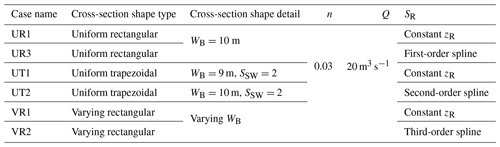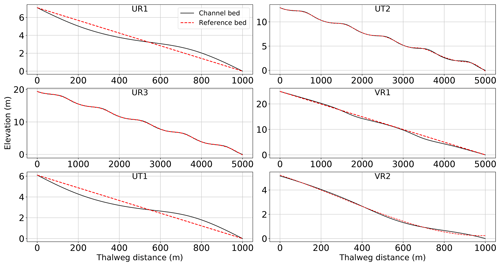Figure 5Channel bed elevation (z0) and reference bed elevation (zR) for six test cases from .

## 3.6 Test cases – synthetic channel reach

The analytical test cases above are designed to show that the RS method does not introduce approximations that affect the smooth solution. However, the true power of the RS method is in solutions of non-smooth bathymetry in which most models using h0 and S0 have difficulty converging. To illustrate this aspect, we use a simple river reach with a randomly perturbed (discontinuous) bathymetry at various scales. As there are no analytical solutions for these tests, we use the HEC-RAS model for comparison. The simulations use time-invariant boundary conditions with geometry defined by trapezoidal cross sections of uniform side slope, as detailed in Table 2. The channel bed offset (hR) and thalweg slope (S0) are illustrated in Fig. 8. The flow boundary conditions provide for a mild slope with a water surface profile that can be classified as an M1 gradually varying flow.

Case 1 is the baseline smooth channel with a uniform slope over the entire reach length. Cases 2 through 5 have a synthetic geometry developed by random perturbations of the bottom elevation of the baseline reach. Cases A and B have the identical smooth geometry to Case 1 but use different reference slopes for RS tests. The synthetic channel test reach is 1.58 km in length discretized into 80 uniform computational nodes with 79 channel segments (20 m per segment). The trapezoidal cross sections each have a 10.0 m bottom width and 63.4 sidewall slopes. Bottom roughness is fixed by a Manning's n value of 0.04 for all segments.

Table 2Configuration of synthetic channel reach test cases: α is used in Eq. (20) for random perturbation of the baseline Case 1 geometry; hR is the bed offset based on Fig. 4 with parentheses indicating upper and lower limits of randomized geometry values over the nonuniform test reach; S0 is the range of the thalweg slope for z0 from Eq. (20); and SR is the selected uniform reference slope.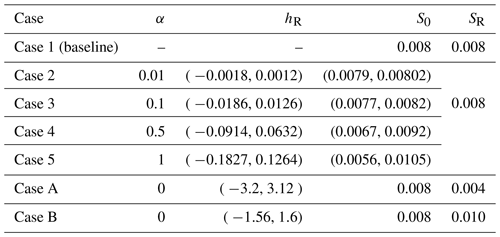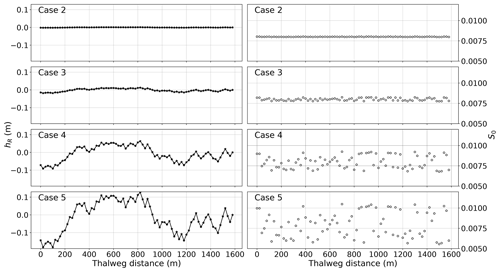Figure 8Channel bottom offset (hR) and physical thalweg slope (S0) for synthetic test cases 2–5 that are random perturbations of the baseline smooth S0=0.008 of Case 1.

To develop the random perturbations of the bottom in the synthetic test cases, we begin with Case 1 (baseline) using a uniform ${S}_{\mathrm{0}}^{\left[\mathrm{1}\right]}=\mathrm{0.008}$ over the entire reach length. Here the superscript in square brackets indicates the case identifier. The set of bottom elevations for Case 1 are ${z}_{\mathrm{0}}^{\left[\mathrm{1}\right]}\left(x\right)$, which are smooth and linearly decreasing over the reach length. Cases 2–5 are similar channels with perturbed bottom elevations set by

$\begin{array}{}\text{(20)}& {z}_{\mathrm{0}}^{\left[c\right]}\left(x\right)={z}_{\mathrm{0}}^{\left[\mathrm{1}\right]}\left(x\right)+{\mathit{\alpha }}^{\left[c\right]}H\left(x\right):c\in \left\{\mathrm{2},\mathrm{3},\mathrm{4},\mathrm{5}\right\},\end{array}$

where H(x) is a set of random-generated numbers within the range of $-\mathrm{0.126}\le H\left(x\right)\le \mathrm{0.183}$. The upper and lower limits of H(x) were selected to prevent the occurrence of a locally adverse slope – such conditions can be handled by SPRNT-RS but can cause convergence problems for some models. The α[c] is a magnitude to generate a range of bottom displacements with ${\mathit{\alpha }}^{\left[c\right]}\in \left\{\mathrm{0.01},\mathrm{0.1},\mathrm{0.5},\mathrm{1.0}\right\}$ for $c\in \left\{\mathrm{2},\mathrm{3},\mathrm{4},\mathrm{5}\right\}$, respectively. Cases 2–5 set the reference bottom elevations exactly equal to the baseline Case 1 physical bottom elevations; i.e.,

$\begin{array}{}\text{(21)}& {z}_{\mathrm{R}}^{\left[c\right]}\left(x\right)={z}_{\mathrm{0}}^{\left[\mathrm{1}\right]}\left(x\right):c\in \left\{\mathrm{2},\mathrm{3},\mathrm{4},\mathrm{5}\right\}.\end{array}$

Thus, the SPRNT-RS simulations for cases 2–5 use uniform SR over the reach such that the bed offset (hR) represents the physical geometric perturbations. Noting from Eq. (13) that hR is the difference between the physical bottom (${z}_{\mathrm{0}}^{\left[c\right]}$) and the reference bottom (${z}_{\mathrm{R}}^{\left[c\right]}$), substituting the above relationships gives

$\begin{array}{}\text{(22)}& {h}_{\mathrm{R}}^{\left[c\right]}\left(x\right)={\mathit{\alpha }}^{\left[c\right]}H\left(x\right).\end{array}$

For synthetic test cases A and B in Table 2, the actual channel bottom slopes are set to uniform values equivalent to Case 1; that is ${S}_{\mathrm{0}}^{\left[A\right]}={S}_{\mathrm{0}}^{\left[B\right]}={S}_{\mathrm{0}}^{\left[\mathrm{1}\right]}=\mathrm{0.008}$ with identical thalweg elevations of ${z}_{\mathrm{0}}^{\left[\mathrm{1}\right]}\left(x\right)$. However, the reference slopes for these cases are set to smaller and greater uniform values: ${S}_{\mathrm{R}}^{\left[A\right]}=\mathrm{0.004}$ and ${S}_{\mathrm{R}}^{\left[B\right]}=\mathrm{0.010}$. These two cases demonstrate that the RS method generates the same numerical solution as baseline Case 1 (solved at S0) when SR is set to an arbitrary value.

Forcing for all seven test cases is a constant inflow boundary of 283 m3 s−1 applied at the furthest upstream node. The downstream boundary condition is 5.0 m depth, which is a subcritical flow based on a normal depth of 4.95 m for S0=0.008 and the inflow rate. Because a subcritical boundary condition allows upstream wave reflections, the downstream boundary was enforced at the end of a 180 m (9 node) buffer domain, which was adequate for reducing upstream wave propagation in unsteady flow solutions. Simulation results are reported after the models have reached a steady state and all oscillations associated with the initial conditions have dissipated. Solutions for the buffer segment are not included in the analyses below.

## 3.7 Test case – Waller Creek study site

The main stem of Waller Creek in Austin, Texas, USA, is used to examine the performance of the RS method for more complex conditions. The main stem of the creek drains an urban watershed of 14.3 km2 with total length of 10.7 km for the area illustrated in Fig. 9. Bathymetric survey data are available courtesy of the city of Austin (Fig. 10). The bathymetric data set includes 327 surveyed cross sections with spacing intervals ranging from 2.5 up to 178 m (mean of 33.5 m). The Manning's n of the channel (based on the city of Austin computations) varies from 0.02 to 0.06 throughout the system. The SPRNT model, in both its original and RS form, has numerical stability issues associated with close cross-section spacing. Arguably, these issues are related to sharp changes in A that lead to non-smooth source terms despite the RS method; however, this issue requires further investigation. Similar numerical instability behavior can also be found in the HEC-RAS unsteady model and also causes divergent solutions. For the present work, we discarded 36 cross sections (11 % of the data set) that were closer than 10 m and merged these short reach lengths with the adjacent sections. An additional three cross sections were discarded and some channel roughness values were modified as they caused numerical instability in the HEC-RAS unsteady simulation (see Appendix for details). The resulting data set is 288 cross sections with spacing ranging from 10.1 to 184.9 m. The mean cross-section spacing is 37.2 m with a total reach length of 10.7 km. To limit our focus to subcritical flow, our analyses consider only the upper 8.3 km of the main reach (210 out of 288 cross sections), which eliminates a series of step-pool transcritical elements in the downstream channel in which the HEC-RAS solution is strongly influenced by the ad hoc local partial inertia (LPI) algorithm . The smoothing introduced by LPI makes it difficult to draw conclusions from a comparison between SPRNT-RS and HEC-RAS across transcritical locations.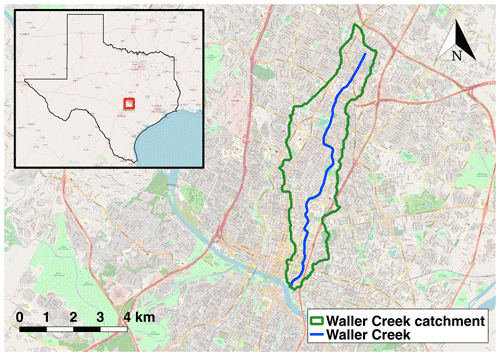Figure 9Main stem of Waller Creek and catchment in Austin (Texas, USA). Basemap © by Open Geospatial Consortium (OGC) Web Mapping Service (WMS).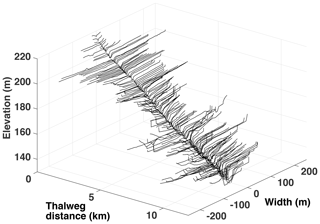Figure 10Surveyed cross sections of Waller Creek. Only 140 out of 327 cross sections are shown for clarity. Elevations are relative to mean sea level. Data courtesy of the city of Austin.

A time-invariant upstream inflow boundary condition is set to 25 m3 s−1 at the headwater cross section. To minimize the influence of subcritical reflections from the upstream inflow boundary, the first 10 computational nodes at the upstream are not included in the results analysis. Lateral inflows are set to zero for all test cases. A 300 m buffer section is added downstream of the test domain to reduce the influence of reflections from the downstream boundary condition. This buffer section uses the same cross section as the final downstream section of the data set with a bed slope (S0) of 0.0033 and Manning's n of 0.04. The buffer section has a normal depth of 0.76 m at the 25 m3 s−1 inflow rate. The downstream boundary condition at the end of the buffer section is 0.7 m depth, which is subcritical and implies an M2 gradually varying drawdown in the vicinity of the outflow. These geometry and boundary conditions are identically applied to both SPRNT-RS and HEC-RAS models.

The thalweg elevation, z0(x), and the reference elevation, zR(x), of the RS method (as determined by the approximate spline fit described above) for Waller Creek are shown in Fig. 11a. The z0 and zR are visually similar with the former being somewhat more noisy. The elevation data sets provide similar overall reach slopes (uppermost cross section to lowermost cross section) of 0.0074 and 0.0077, respectively. Note that the approximate B-spline technique for generating zR(x) does not force the overall reach slope to be identical. Because zR(x) is mathematically arbitrary, there is no need to force an exact match. Although z0(x) and zR(x) are similar in Fig. 11a, S0(x) from the raw data is discontinuous and varies over a wide range (up to 4 times the reach slope), as illustrated in Fig. 11b. Note that S0(x) also includes negative slopes (i.e., adverse gradient sections) which can cause convergence problems for some numerical solvers. In contrast, as shown in Fig. 11c, the approximate cubic B-spline used to generate SR(x) from zR(x) provides a reference slope that is everywhere smooth and positive and remains close to the overall reach slope of 0.008. The slope range and model nomenclature for the Waller Creek test cases are provided in Table 3. The Lipschitz smoothness of S0 versus SR can be better understood by evaluating the gradient of the slope, i.e., the second derivative of z0 and zR, as shown in Fig. 11d. The S0 formulation clearly lacks smoothness in the higher derivative.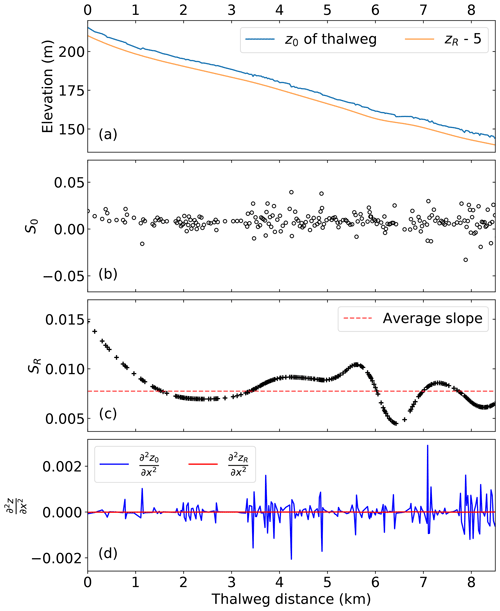Figure 11Waller Creek bottom elevation and slope: (a) z elevation, with 5 m subtracted from zR for clarity; (b) S0 thalweg slope between cross sections; (c) SR smoothed bottom slope; and (d) second derivative of z0 and zR. Note the y-axis scaling in (c) has reduced limits compared to (b) to better show the smoothness achieved by the spline fit.

## 3.8 Analysis methods

To evaluate the performance of the RS method relative to conventional formulations, four depth-based indicators are employed, as described below. For these definitions, the control (superscript [C]) is the solution for the analytical test case and HEC-RAS results for the synthetic channel and Waller Creek test cases. Note that the synthetic tests use unsteady HEC-RAS, whereas the Waller Creek study uses comparisons to both steady and unsteady versions of the model. The test case (superscript [T]) is always the SPRNT-RS simulation. These measures can be considered error metrics for the comparison to the analytical solutions and difference metrics for model–model comparisons.

1. Normalized difference (ρ). A nondimensional index to describe the local difference in depth can be defined as

$\begin{array}{}\text{(23)}& {\mathit{\rho }}^{\left[T:C\right]}\left(x\right)=\frac{{h}_{\mathrm{0}}^{\left[C\right]}\left(x\right)-{h}_{\mathrm{0}}^{\left[T\right]}\left(x\right)}{{h}_{\mathrm{0}}^{\left[C\right]}\left(x\right)},\end{array}$

where ${h}_{\mathrm{0}}^{\left[C\right]}\left(x\right)$ and ${h}_{\mathrm{0}}^{\left[T\right]}\left(x\right)$ are the local depth solutions from the control and test case results after steady-state conditions are achieved. The normalization scale is the local depth of the control case. Note the denominator is nonzero in the synthetic test case setup because the flow setup is an M1 gradually varying flow.

2. Absolute mean normalized difference (ζ). The mean of the absolute value of ρ(x) over the domain provides an overall nondimensional indicator of the depth error:

$\begin{array}{}\text{(24)}& {\mathit{\zeta }}^{\left[T:C\right]}=\frac{\mathrm{1}}{N}\sum _{x=\mathrm{1}}^{N}\mid {\mathit{\rho }}^{\left[T:C\right]}\left(x\right)\mid ,\end{array}$

where N is the total number of cross sections. We use the absolute value so that positive errors do not cancel negative errors, and ζ is a representative scale of the discrepancy between models.

3. Mean absolute error (MAE). The overall dimensional error is characterized as

$\begin{array}{}\text{(25)}& \text{MAE}=\frac{\mathrm{1}}{N}\sum _{x=\mathrm{1}}^{N}\mid {h}_{\mathrm{0}}^{\left[C\right]}\left(x\right)-{h}_{\mathrm{0}}^{\left[T\right]}\left(x\right)\mid ,\end{array}$

and the nondimensional form of overall error is

$\begin{array}{}\text{(26)}& \begin{array}{rl}\text{MAE}& \phantom{\rule{0.25em}{0ex}}\text{(nondimensional)}\\ & =\frac{\mathrm{1}}{N}\sum _{x=\mathrm{1}}^{N}\mid \frac{{h}_{\mathrm{0}}^{\left[C\right]}\left(x\right)-{h}_{\mathrm{0}}^{\left[T\right]}\left(x\right)}{{h}_{\mathrm{0}}^{\left[C\right]}\left(x\right)}\mid .\end{array}\end{array}$
4. Root mean square error (RMSE). A standard dimensional measure of the squared error is

$\begin{array}{}\text{(27)}& \text{RMSE}=\sqrt{\frac{\mathrm{1}}{N}\sum _{x=\mathrm{1}}^{n}\left({h}_{\mathrm{0}}^{\left[C\right]}\left(x\right)-{h}_{\mathrm{0}}^{\left[T\right]}\left(x\right){\right)}^{\mathrm{2}}}.\end{array}$

The nondimensional form of RMSE is computed by the following equation:

$\begin{array}{}\text{(28)}& \phantom{\rule{0.33em}{0ex}}\begin{array}{rl}\text{RMSE}& \phantom{\rule{0.25em}{0ex}}\text{(nondimensional)}\\ & =\sqrt{\frac{\mathrm{1}}{N}\sum _{x=\mathrm{1}}^{n}\left(\frac{{h}_{\mathrm{0}}^{\left[C\right]}\left(x\right)-{h}_{\mathrm{0}}^{\left[T\right]}\left(x\right)}{{h}_{\mathrm{0}}^{\left[C\right]}\left(x\right)}{\right)}^{\mathrm{2}}}.\end{array}\end{array}$

Both the MAE and RMSE are also reported in nondimensional form where the normalization scale is presented.

4 Results

## 4.1 Analytical test cases

The water surface elevations for the analytical solutions and SPRNT-RS simulations are shown in Fig. 12. Visually, the analytical and simulated results across all six cases are identical. Error metrics following Sect. 3.8 are provided in Table 1. The normalized differences (ρ) are less than 1 % and are consistent with absolute errors of O(10−3) m, which are negligible compared with the water depths ≥1 m. The spatial distributions of the normalized error are shown in Fig. 13. By comparing this figure with Fig. 5, it can be seen that ρ(x) fluctuates with the change in bed slope. Similar behavior can also be found for model results reported in .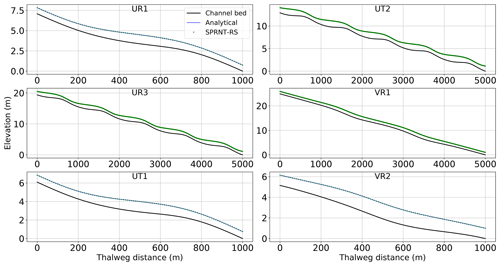Figure 12Comparison between simulated water surface elevation from SPRNT-RS and analytical solution for analytical test cases of .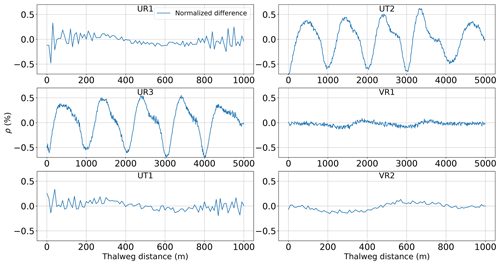Figure 13Spatial distribution of normalized difference (ρ) for analytical test cases of .

Table 4Difference measures using Eqs. (23)–(28) for analytical test cases of . Nondimensional MAE and RMSE are shown in parentheses.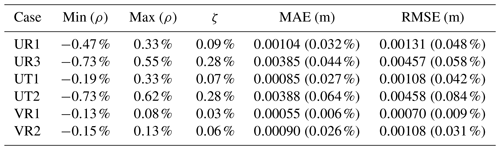## 4.2 Synthetic test cases

Results for the baseline synthetic test, Case 1, are shown in Fig. 14. The SPRNT-RS method produces visually the same solution to HEC-RAS with zR=z0. Similarly, the comparison of model results for depth (h0) for test cases 2–5 are visually indistinguishable, as shown in the left column of Fig. 15. The quantitative difference measures for the synthetic tests are provided in Table 5, and the spatial distributions of ρ(x) are illustrated in the right column of Fig. 15. Values for ρ(x) in cases 2 and 3 are slightly below zero (≈0.02 %) over the entire domain, indicating the SPRNT-RS solution has a slightly higher water surface than the HEC-RAS solution for small perturbations in the bed slope. With the increased bottom perturbations in cases 4 and 5, the ρ(x) range is larger (and includes a positive range), but the bounding values are still trivial. The ζ and RMSE measures show that the nondimensional and dimensional overall differences are small. The MAE and RMSE climb slightly with the increasing hR for cases 2 through 5 but remain below 3 mm. These depth RMSE values are negligible compared with the normal depth (4.95 m) of the baseline and well within reasonable truncation error differences for solvers using different numerical techniques. The model–model comparisons for test cases A and B also have trivial errors (Table 5), and further results are not shown as they are visually identical to those for baseline Case 1 illustrated in Fig. 14. Note that the RMSE for both cases is identical to Case 1, which indicates the solution for the SPRNT-RS method with SRS0 is very close to the baseline solution with SR=S0.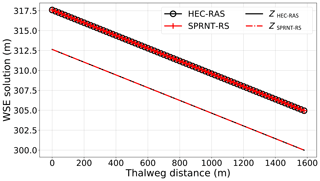Figure 14Simulated profile of water surface elevation (upper line) and channel bottom (lower line) for synthetic test Case 1 using SPRNT-RS (red) and unsteady HEC-RAS (black).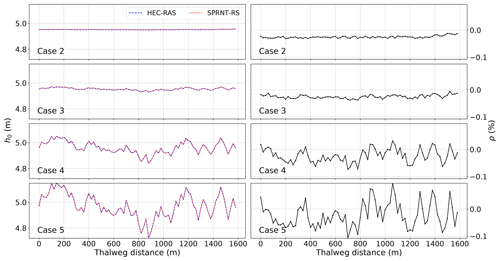Figure 15Water depth, h0, (left column) and normalized difference, ρ, (right column) for synthetic test cases with perturbed bathymetry.

Table 5Difference measures between SPRNT-RS and HEC-RAS using Eqs. (23)–(28) for synthetic test cases. Nondimensional MAE and RMSE are shown in parentheses.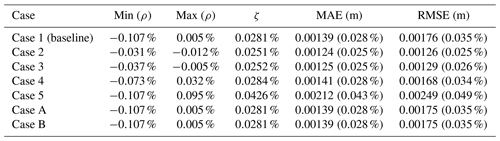## 4.3 Waller Creek test case

Waller Creek has been simulated with SPRNT-RS (denoted as WCRS in the following figures), the HEC-RAS unsteady solver (WCHEC-U), and the HEC-RAS steady solver (WCHEC-S). Figure 16 shows water surface elevations for SPRNT-RS and unsteady HEC-RAS. For clarity, the upper 40% of the domain (which has similar good behavior) is not shown. Figure 17 shows the spatial distribution of the normalized difference, ρ, for these simulations. The differences are roughly within ±4 % across the entire domain. The maximum and minimum difference both occur at two adjacent nodes close to 7800 m with 4.14 % and −3.07 %, respectively. Figure 18 provides a similar comparison of water surface elevations between SPRNT-RS and the steady HEC-RAS case. The results are visually quite similar to the comparison with unsteady HEC-RAS. A direct comparison of surface elevations for unsteady and steady HEC-RAS does not provide any further insight and is omitted for brevity. However, to quantitatively evaluate the differences between SPRNT-RS and HEC-RAS, it is useful to compute difference measures between the unsteady and steady HEC-RAS models themselves, as well as the differences between SPRNT-RS and both models, as provided in Table 6. Overall, the SPRNT-RS result have marginally better consistency with unsteady HEC-RAS than with steady HEC-RAS. Of greater importance is that the behavior of SPRNT-RS relative to unsteady HEC-RAS has the same order of differences as the comparison of unsteady HEC-RAS to steady HEC-RAS. These results imply that the differences between SPRNT-RS and unsteady HEC-RAS are reasonable for the different numerical methods given the geometric variability of Waller Creek.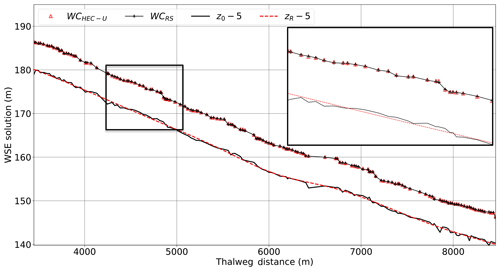Figure 16Comparison of SPRNT-RS to unsteady HEC-RAS for water surface elevations in Waller Creek simulations with expanded detail to show similarities. For clarity, 5 m is subtracted from the channel z elevations.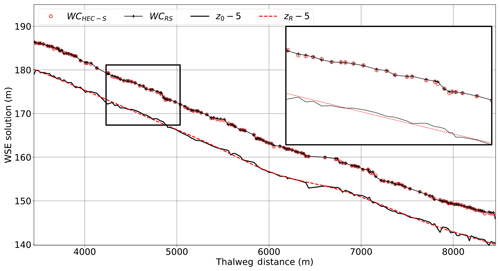Figure 18Comparison of SPRNT-RS to steady HEC-RAS for water surface elevations in Waller Creek simulations with expanded detail to show similarities. For clarity, 5 m is subtracted from the channel z elevations.

Table 6Difference metrics for Waller Creek simulation results. Nondimensional MAE and RMSE are shown in parentheses.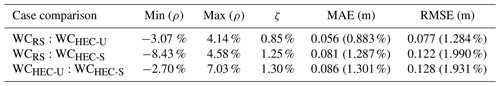5 Discussion

## 5.1 Validation of the RS method

The RS method is a simple algebraic transformation of the governing equations, and the answer to the principal question “does it work” is implied by our inability use the baseline SPRNT model (with S0) as a control model (see Sect. 3.4). Invariably, discontinuous topography for SPRNT without RS caused either an oscillatory solution or numerical instability. In contrast, both HEC-RAS (using η) and SPRNT-RS (using SR) provide stable, non-oscillatory solutions.

The analytical results in Sect. 4.1, supplemented by additional results in , validate the SPRNT-RS method for the simulation of smoothly varying channel morphologies that are Lipschitz continuous at the discretization scale. We have experimented with both uniform and splined SR for these tests. For both types of simulations, we observe errors relative to physical experiments that are comparable or smaller than those shown in the numerical validation studies of . These results imply that the transformation from the conventional h0 and S0 form of the SVEs to the ha and SR form of Eq. (17) is a valid algebraic step that can be implemented in a numerical solver and is an alternative for representing smooth geometries.

The synthetic test cases in Sect. 4.2 serve two purposes. Firstly, cases A and B compared to baseline Case 1 show that the numerical solution does not depend on a particular choice of SR. The arbitrary selection of an SRS0 results in identical solutions to SR=S0. Secondly, the synthetic test cases show that the SPRNT-RS method can be applied with non-smooth geometry at the discretization scale (i.e., random perturbations of the physical bottom slope), which caused non-convergent behavior in the baseline SPRNT model. As a control, we have compared SPRNT-RS with the accepted HEC-RAS model that remains stable for these test cases as it is solved with the piezometric pressure gradient rather than splitting into S0 and the gradient of h0. The results indicate that SPRNT-RS provides numerical solutions that are nearly identical to HEC-RAS for the non-smooth geometry test cases. Thus, using a Lipschitz smooth SR provides a stable numerical solution for non-smooth geometry without altering the physical representation of non-smooth geometry.

The Waller Creek test case in Sect. 4.3 provides a more challenging comparison of SPRNT-RS to HEC-RAS. For this test case, the geometry discontinuities include adverse slopes and local S0 that are ±400 % of the reach-average slope, which contrasts with perturbations of ±30 % used in the synthetic test cases of Sect. 4.2. Again, SPRNT-RS is shown to be close to the unsteady HEC-RAS solution. The model differences are within reasonable ranges, as illustrated by the fact that they are similar to the differences between HEC-RAS steady and unsteady versions. Nevertheless, it remains possible that the minor differences between HEC-RAS and SPRNT-RS are caused by a latent defect in coding the RS method or in SPRNT itself, but it is difficult to envision how such a defect could occur without also appearing in the analytical and synthetic test cases. A simpler and more compelling explanation is with the linear approximations used in unsteady HEC-RAS that are not present in SPRNT-RS. Specifically, notes that for computational efficiency and to reduce “troublesome convergence problems at discontinuities in the river geometry,” the unsteady HEC-RAS code uses a linearization technique developed by and Chen (1973) – note that the latter document is cited by but was not available to us. It seems likely that strong geometry discontinuities in the Waller Creek test case would be affected by this linearization, which arguably would lead to artificial smoothing of the water surface profile by HEC-RAS. Unfortunately, we do not have direct access to the HEC-RAS code and thus rely on the discussion of HEC-RAS stability in the literature and the methodology in HEC-RAS manuals (Brunner2016a, b).

## 5.2 Why not just use $\partial \mathit{\eta }/\partial x$?

One might wonder whether SR or S0 is at all necessary when we could clearly just retain $\partial \mathit{\eta }/\partial x$ in the SVEs rather than using any split form. To understand the value of SR, it is worth considering why S0 is presently used. We have not been able to determine exactly when S0 was first used with the SVEs, but from a hydrology viewpoint S0 provides consistency between kinematic wave solutions (which use S0=Sf) and the SVEs. Thus including S0 is a logical step when considering reduced-physics approaches . Arguably, a well-chosen SR that matches the large-scale S0 will serve the same purpose. The S0 approach is also favored in models that are built on a “conservative” SVE form in which the hydrostatic pressure portion of the piezometric head gradient is abstracted into the advective gradient term (e.g., Sanders2001; Kesserwani2013). For these model, the advantage of the S0 form is that, when S0 equals 0 and Sf equals 0, the momentum equation can be written as a classic 1D homogenous advection equation, which is mathematically appealing. Our work in progress indicates that the SR approach could be similarly adapted for a conservative form of the SVEs, but this issue remains speculative.

Although the utility and simplicity of the η approach is obvious, it has a key disadvantage when applied in large-scale simulations. Over large distances the free surface is monotonically increasing upstream, which has consequences for employing implicit or semi-implicit numerical solutions in a continental river dynamics framework (Hodges2013). Briefly, when modeling a river system from an estuary (η∼0 m) to mountain headwaters (η∼103 m), the solution variable, η, nominally covers 3 orders of magnitude. Furthermore, as local variations on the order of 10−2 m affect the hydrostatic pressure gradient, the solution of η requires precision over at least 5 orders of magnitude – i.e., a stiff numerical solution that can be difficult to converge for either a linear or nonlinear solver. Thus, splitting $\partial \mathit{\eta }/\partial x$ into a down-slope body force (SR) and a local residual ($\partial {h}_{\mathrm{a}}/\partial x\right)$ is effectively removing a large-scale gradient from the solution variables, which will generally improve numerical behavior.

Despite the above disadvantages, the η form retains some advantages in creating conservative finite-volume formulations of the Saint-Venant equations (Hodges2019). Arguably, such methods should be confined to explicit time-marching schemes or localized solutions, in which η covers a smaller range, and the RS method should be preferred for larger systems.

## 5.3 RS advantages and limitations

The fundamental difference between the SPRNT-RS approach and most, if not all, conventional models (including unsteady HEC-RAS) is that our method algebraically revises the Saint-Venant equations to exactly accommodate discontinuous geometry while maintaining a smooth source term, whereas other models typically introduce ad hoc changes (e.g., linearization) to provide stable and faster numerical behavior when discontinuities are likely to cause numerical instabilities. These differences in the governing equations can be expected to cause differences in the solution – especially where nonlinear terms are strong. The present scope is limited in that only a single model was modified (SPRNT/SPRNT-RS) and only a single model (HEC-RAS) was used as an external control. The validity of the underlying algebraic transformation in the RS method has been demonstrated by these tests; however, it remains to be seen how implementing the RS approach in other models – particularly well-balanced models – might alter residual errors, convergence rates, and computational performance. We are interested in collaborating with other researchers who have access to and familiarity with source code of candidate well-balanced models.

An important limitation to the present work is that we focus solely on subcritical flow. The Preissmann scheme used in the underlying SPRNT model is known to exhibit instabilities with transcritical flows , which can be suppressed with the ad hoc local partial inertia (LPI) scheme of . Our preliminary work (not shown) indicates that the RS approach can stabilize the Preissmann scheme without using LPI, but further work is required to test and validate the RS method for transcritical and supercritical flows.

Overall, the RS method can ensure the Lipschitz smoothness of slope representation in the momentum source term (without smoothing geometry), thus reducing one source of oscillatory or unstable behavior in numerical solutions. However, application of the RS method is not without some limitations. Although the switch from S0 to SR is algebraically exact, the application of the RS method requires some method to select the distributed zR(x) and to determine SR(x). Poor selection of zR can theoretically result in non-smooth SR. In the present work, the profile of zR in the Waller Creek case is generated by the cubic B-spline technique, which is controlled by the number of knots and their spacing. In general, the distance between knots must be longer than the spacing between cross sections so that the generated SR is smooth at the model's discretization scale. It is not clear that a mathematical “optimum” for selection of knots will necessarily exist, but there are likely (unknown) practical limits on knot selection spacing for “adequate” smoothness of zR(x). Our results indicate that approximating cubic B-splines is adequate for producing smooth zR for the tested geometries and that the solutions are robust for the selection of zR as long as SR is smooth . However, it is likely there are limitations to applying the RS method in large-scale river network simulations that will make it difficult to use a simple globally applied knot spacing. Such networks might consist of 104 to 105 reaches spanning wide geographical regions with a variety of topology and inconsistent data availability. Some reaches may have well-defined cross sections at close spacing, other reaches might be poorly documented (Hodges2013). Thus, it seems likely that a method for automatically generating approximating splines (or some other form of smoothing) would be useful, but such an advance arguably requires a method for quantitatively evaluating the goodness of a particular set of zR(x), which remains an open question. We speculate that simple window filtering techniques may be adequate for river databases such as NHDplus (United States National Hydrography Dataset Plus), but further investigation and examination are needed to better understand the interplay between the smoothing scales and the numerical solution using the RS method for large networks.

This study has implemented RS only in the SPRNT code, as discussed in more detail in Sect. 3. The baseline governing equations for SPRNT are of the form of Eq. (2), the so-called “nonconservative” form, which simply means that the entirety of the hydrostatic pressure gradient is effectively a source term, in contrast to “conservative” equations such as the Cunge–Liggett form , in which a portion of the hydrostatic pressure gradient is abstracted to the advection term. Although it remains to be shown in future work, the algebraic transformation implied in Eq. (4) can arguably be applied in the Cunge–Liggett form or any other conservative form of the SVEs. Similarly, the fundamental algebraic transformation to SR and $\partial {h}_{\mathrm{a}}/\partial x$ will be equally valid in any finite-volume method using S0 and h0.

The greatest barrier to the adoption of the RS method in an existing model is likely the need to rewrite the geometry functions to accept ha, hR, and zR in place of h0 and z0. The difficulties involved in this effort depend on whether or not the model geometry functions are sufficiently isolated from the main solution algorithm. Indeed, we can imagine codes where the geometry functions are essentially dispersed throughout and require extensive effort to alter, debug, and verify.

## 5.4 The future for RS methods

The RS method as introduced above might be just a starting point. Although the present work focused on the nonconservative form, the concepts presented herein will likely be effective in addressing the well-balanced problem for conservative forms as reviewed in and . Furthermore, the algebra in the RS demonstration above leads to the conjecture that the method could be extended to 2D reference slopes for bathymetry in 2D or 3D models. Undoubtedly there are unknown numerical challenges in extending to higher dimensions – particularly in ensuring a 2D spline function is adequately spaced to ensure smoothness – but there does not appear to be any fundamental conceptual difficulty in such efforts.

6 Conclusions

The reference slope (RS) method introduces a new form of the Saint-Venant equations for 1D river flow. The advantage of the RS method is that it ensures the body force (slope) source term is smooth and cannot destabilize the numerical solution. The RS method introduces the concept of an arbitrary smooth reference elevation, zR(x), with computed reference slopes, SR(x), and associated depths, ha(x). These geometries are algebraically related to the traditional channel thalweg elevation, depth, and bottom slope (${z}_{\mathrm{0}},{h}_{\mathrm{0}},{S}_{\mathrm{0}}$) used in many models. The RS method is implemented in an open-source Saint-Venant solver as SPRNT-RS. In this study, SPRNT-RS was compared to both analytical solutions and the conventional HEC-RAS model for synthetic test reaches and an urban creek for subcritical flows. The model–model comparisons are within the expected truncation error for both the analytical and synthetic test cases and within acceptable differences for simulating flow through the complex geometry of an urban creek. The slightly larger simulation differences in the urban creek test case are likely due to ad hoc linearization algorithms used in HEC-RAS that do not appear in SPRNT-RS.

As discussed in the Sect. 2, when faced with non-smooth geometries in a channel reach, prior researchers have resorted to limiting or smoothing discontinuous source terms or employing numerical techniques that mitigate oscillatory/unstable numerical behaviors. In contrast, the new RS method transforms a discontinuous bottom slope source term into a smooth expression without losing either complexity in the geometry or introducing ad hoc smoothing of the geometry, the numerical method, or the solution. An important advantage of the RS method is that it is entirely mechanical, requiring only the selection of control knot spacing for the approximating spline at some length scale larger than the cross-section spacing. That is to say that RS does not require the model designer or user to introduce smoothing thresholds or ad hoc algorithm bounds. As such, we believe the RS method could be particularly valuable as we move from fine-resolution reach-scale modeling to large-scale continental river dynamic simulations (Hodges2013) or develop massively parallel stormwater network models for megacities

The RS method is not specific to SPRNT but can be adapted to any Saint-Venant solver that uses a bottom slope (S0) term in the discretization. The mathematical change is conceptually trivial, but the actual effort depends on how cross-section geometry is embedded in the code. The code for both SPRNT and SPRNT-RS are available under open-source license at GitHub (Liu2014).

Appendix A: Geometry adjustments for stable unsteady HEC-RAS simulations

As discussed in Sect. 3, the stability of the SPRNT-RS simulations for Waller Creek was ensured by merging 36 computational elements in which the cross-section spacing was closer than 10 m. This minimum spacing cutoff was selected as being well below the median spacing of 28.6 m and mean spacing of 37.7 m and proved adequate for ensuring SPRNT-RS stability in the tested simulations. Unfortunately, stability of unsteady HEC-RAS required the further removal of three cross-section elements (shown in Fig. A1) and reducing Manning's n at six additional cross sections (listed in Table A1). Selecting these changes was a matter of art rather than science as we could not identify clear criteria for cross-section removal or Manning's n adjustments for HEC-RAS – other than that these locations appeared to be where instabilities appeared in unsteady HEC-RAS model runs. Although SPRNT-RS could run without these changes, for consistency in the model comparisons the geometry of the SPRNT-RS model was modified to exactly match the adjusted geometry required for the HEC-RAS model.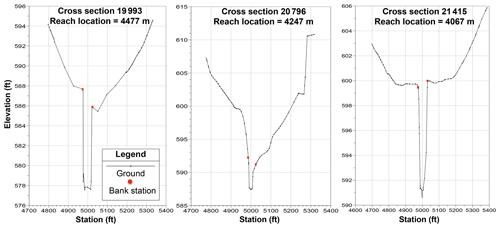Figure A1Cross sections removed from Waller Creek data set to provide numerical stability of unsteady HEC-RAS.

Table A1Modified Manning's n for cross-section stations in Waller Creek data set to provide numerical stability of unsteady HEC-RAS.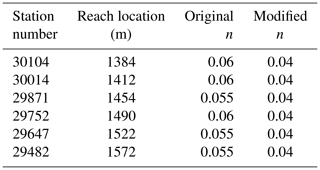Appendix B: Notation
 A cross-section area (m2) g gravitational acceleration (ms−2) h0 water depth (m) ha associated water depth (m) hR reference height (m) n Gauckler–Manning–Strickler roughness (${\text{m}}^{-\mathrm{1}/\mathrm{3}}\text{s}$) Pw wetted perimeter (m) Q volumetric flow rate (m3s−1) qℓ flow rate per unit length through channel sides (m2 s−1) S0 channel bottom slope Sf channel friction slope SR channel reference slope SSW channel sidewall slope t time (s) W channel width (m) WB channel bottom width ((m)) x along-channel spatial coordinate z0 channel bottom elevation (m) zR reference elevation (m) α bottom displacement coefficient ϵ second derivative of water surface elevation (m−1) η water surface elevation (m) ρ normalized difference between results ζ absolute mean normalized difference (AMND)
Code availability

The complete code for the reference slope module and SPRNT is available at Github (https://github.com/frank-y-liu/SPRNT, https://doi.org/10.5281/zenodo.3978737, ).

Data availability

All test case files and results have been uploaded to a public repository under the Texas Data Repository and can be accessed through https://doi.org/10.18738/T8/BXJBF5.

Author contributions

CWY and BRH designed and performed the experiments, analyzed the data, and wrote the paper. FL wrote the code for the reference slope method.

Competing interests

The authors declare that they have no conflict of interest.

Disclaimer

This research has not been formally reviewed by the U.S. Environmental Protection Agency (EPA). The views expressed in this document are solely those of the authors and do not necessarily reflect those of the agency. The EPA does not endorse any products or commercial services mentioned in this publication.

Acknowledgements

The authors would like to thank Fernando R. Salas from the U.S. National Water Center for providing assistance on collecting and processing the data for the test cases. The first author, CWY, would also like to thank Alan Plummer Associates, Inc., for providing additional support during the latter stages of this project. Frank Liu would like to acknowledge the support from the Laboratory Directed Research and Development program of Oak Ridge National Laboratory, managed by UT-Battelle, LLC, for the U.S. Department of Energy.

Financial support

This research has been supported by the U.S. National Science Foundation (grant no. CCF-1331610) and the U.S. Environmental Protection Agency (cooperative agreement no. 83595001).

Review statement

This paper was edited by Roberto Greco and reviewed by two anonymous referees.

References

Aggett, G. R. and Wilson, J. P.: Creating and coupling a high-resolution DTM with a 1-D hydraulic model in a GIS for scenario-based assessment of avulsion hazard in a gravel-bed river, Geomorphology, 113, 21–34, https://doi.org/10.1016/j.geomorph.2009.06.034, 2009. a

Brunner, G. W.: HEC-RAS, River Analysis System Reference Manual, U.S. Army Corps of Engineers, Hydrologic Engineering Center, Technical Report CPD-69, Davis, California, USA, available at: [13:43] Viola Zierenberg https://www.hec.usace.army.mil/software/hec-ras/documentation/HEC-RAS 5.0 Reference Manual.pdf (last access: 10 August 2020), 2016a. a, b, c

Brunner, G. W.: HEC-RAS, River Analysis System User's Manual, Version 5.0, U.S. Army Corps of Engineers, Hydrologic Engineering Center, Technical Report CPD-68, Davis, California, USA, available at: https://www.hec.usace.army.mil/software/hec-ras/documentation/HEC-RAS 5.0 Users Manual.pdf (last access: 10 August 2020), 2016b. a, b

Burguete, J., Garcia-Navarro, P., Murillo, J., and Garcia-Palacin, I.: Analysis of the Friction Term in the One-Dimensional Shallow-Water Model, Journal of Hydraul. Eng.-ASCE, 133, 1048–1063, https://doi.org/10.1061/(ASCE)0733-9429(2007)133:9(1048), 2007. a

Chen, Y. H.: Mathematical Modeling of Water and Sediment Routing in Natural Channels, PhD thesis, Colorado State University, Ft. Collins, CO, 1973. a

Cunge, J. A., Holly, F. M., and Verwey, A.: Practical Aspects of Computational River Hydraulics, Pitman Publishing Ltd, Boston, MA, USA, 1980. a

de Boor, C.: A Practical Guide to Splines, Springer-Verlag, New York Berlin Heidelberg, 2001. a

Decoene, A., Bonaventura, L., Miglio, E., and Saleri, F.: Asymptotic derivation of the section-averaged shallow water equations for natural river hydraulics, Math. Mod. Meth. Appl. S., 19, 387–417, https://doi.org/10.1142/S0218202509003474, 2009. a

Di Baldassarre, G.: Floods in a Changing Climate: Inundation Modelling, International Hydrology Series, Cambridge University Press, Cambridge, UK, https://doi.org/10.1017/CBO9781139088411, 2012. a

Fread, D. L., Jin, M., and Lewis, J. M.: An LPI numerical implicit solution for unsteady mixed-flow simulation, in: North American Water and Environment Congress, vol. 96, pp. 49–72, 1996. a, b

Gichamo, T. Z., Popescu, I., Jonoski, A., and Solomatine, D.: River cross-section extraction from the ASTER global DEM for flood modeling, Environ. Modell. Softw., 31, 37–46, https://doi.org/10.1016/j.envsoft.2011.12.003, 2012. a

Giustarini, L., Matgen, P., Hostache, R., Montanari, M., Plaza, D., Pauwels, V. R. N., De Lannoy, G. J. M., De Keyser, R., Pfister, L., Hoffmann, L., and Savenije, H. H. G.: Assimilating SAR-derived water level data into a hydraulic model: a case study, Hydrol. Earth Syst. Sci., 15, 2349–2365, https://doi.org/10.5194/hess-15-2349-2011, 2011. a

Greenberg, J. M. and LeRoux, A.-Y.: A well-balanced scheme for the numerical processing of source terms in hyperbolic equations, SIAM Journal on Numerical Analysis, 33, 1–16, https://doi.org/10.1137/0733001, 1996. a

Hicks, F. E. and Peacock, T.: Suitability of HEC-RAS for flood forecasting, Can. Water Resour. J., 30, 159–174, https://doi.org/10.4296/cwrj3002159, 2005. a

Hodges, B. R.: Challenges in Continental River Dynamics, Environ. Modell. Softw., 50, 16–20, https://doi.org/10.1016/j.envsoft.2013.08.010, 2013. a, b, c

Hodges, B. R.: Conservative finite-volume forms of the Saint-Venant equations for hydrology and urban drainage, Hydrol. Earth Syst. Sci., 23, 1281–1304, https://doi.org/10.5194/hess-23-1281-2019, 2019. a, b, c

Horritt, M. S. and Bates, P. D.: Evaluation of 1D and 2D numerical models for predicting river flood inundation, J. Hydrol., 268, 87–99, https://doi.org/10.1016/S0022-1694(02)00121-X, 2002. a

Iserles, A.: A First Course in the Numerical Analysis of Differential Equations, Cambridge University Press, Cambridge, UK, 1996. a

Kesserwani, G.: Topography discretization techniques for Godunov-type shallow water numerical models: a comparative study, J. Hydraul. Res., 51, 351–367, https://doi.org/10.1080/00221686.2013.796574, 2013. a, b, c, d

Kuiry, S. N., Sen, D., and Bates, P. D.: Coupled 1D-Quasi-2D Flood Inundation Model with Unstructured Grids, J. Hydraul. Eng.-ASCE, 136, 493–506, 2010. a

Liang, Q. and Marche, F.: Numerical resolution of well-balanced shallow water equations with complex source terms, Adv. Water Resour., 32, 873–884, https://doi.org/10.1016/j.advwatres.2009.02.010, 2009. a, b

Liggett, J. A.: Numerical method of solution of the unsteady flow equations, Unsteady Flow in Open Channels, 1, 89–182, 1975. a

Liu, F.: SPRNT: A River Dynamics Simulator, available at: https://github.com/frank-y-liu/SPRNT, last access; 4 September, 2018, 2014. a, b, c

Liu, F. and Hodges, B. R.: Applying microprocessor analysis methods to river network modeling, Environ. Modell. Softw., 52, 234–252, https://doi.org/10.1016/j.envsoft.2013.09.013, 2014. a, b, c

Liu, F. and Yu, C.-W.: frank-y-liu/SPRNT: minor bug fixes (Version v1.3.8), Zenodo, https://doi.org/10.5281/zenodo.3978737, 2020. a

MacDonald, I., Baines, M., Nichols, N., and Samuels, P.: Comparison of some steady state Saint-Venant solvers for some test problems with analytic solutions, Numerical Analysis Report 2/95, Department of Mathematics, University of Reading, available at: http://www.reading.ac.uk/web/files/maths/02-95.pdf (last access: 10 August 2020), 1995. a, b, c, d, e, f, g, h, i, j, k, l

Martinez-Aranda, S., Murrillo, J., and Garcia-Navarro, P.: A 1D numerical model for the simulation of unsteady and highly erosive flows in rivers, Comput. Fluids, 181, 8–34, https://doi.org/10.1016/j.compfluid.2019.01.011, 2019. a

Mejia, A. I. and Reed, S. M.: Evaluating the effects of parameterized cross section shapes and simplified routing with a coupled distributed hydrologic and hydraulic model, J. Hydrol., 409, 512–524, https://doi.org/10.1016/j.jhydrol.2011.08.050, 2011. a

Meselhe, E. and Holly Jr., F.: Invalidity of Preissmann scheme for transcritical flow, J. Hydraul. Eng., 123, 652–655, https://doi.org/10.1061/(ASCE)0733-9429(1997)123:7(652), 1997. a

Morales-Hernández, M., Sharif, M. B., Gangrade, S., Dullo, T. T. , Kao, S.-C., Kalyanapu, A., Ghafoor, S. K., Evans, K. J., Madadi-Kandjani, E., and Hodges, B. R.: High-performance computing in water resources hydrodynamics. Journal of Hydroinformatics, jh2020163, https://doi.org/10.2166/hydro.2020.163, 2020. a

Nujic, M.: Efficient implementation of nonoscillatory schemes for the computation of free-surface flows, J. Hydraul. Res., 33, 101–111, https://doi.org/10.1080/00221689509498687, 1995. a

Preissmann, A.: Progagation des intumescences dans les canaux et rivières, in: Proceedings of First Congress of French Association for Computation, Grenoble, France, 433–442, 1961. a

Samuels, P. G. and Skeels, C. P.: Stability limits for Preissmann's scheme, J. Hydraul. Eng., 116, 997–1012, https://doi.org/10.1061/(ASCE)0733-9429(1990)116:8(997), 1990. a

Sanders, B. F.: High-resolution and non-oscillatory solution of the St. Venant equations in non-rectangular and non-prismatic channels, J. Hydraul. Res., 39, 321–330, https://doi.org/10.1080/00221680109499835, 2001. a, b

Sart, C., Baume, J.-P., Malaterre, P.-O., and Guinot, V.: Adaptation of Preissmann's scheme for transcritical open channel flows, J. Hydraul. Res., 48, 428–440, https://doi.org/10.1080/00221686.2010.491648, 2010. a

Schippa, L. and Pavan, S.: Analytical treatment of source terms for complex channel geometry, J. Hydraul. Res., 46, 753–763, 2008. a

Sharkey, J. K.: Investigating Instabilities with HEC-RAS Unsteady Flow Modeling for Regulated Rivers at Low Flow Stages, MS thesis, University of Tennessee, Knoxville, Tennessee, USA, available at: https://trace.tennessee.edu/utk_gradthes/3183 (last access: 10 August 2020), 2014. a

Singh, J., Altinakar, M. S., and Ding, Y.: Numerical modeling of rainfall-generated overland flow using nonlinear shallow-water equations, J. Hydrol. Eng., 20, 04014089, https://doi.org/10.1061/(ASCE)HE.1943-5584.0001124, 2015. a

Song, L., Zhou, J., Li, Q., Yang, X., and Zhang, Y.: An unstructured finite volume model for dam-break floods with wet/dry fronts over complex topography, Int. J. Numer. Meth. Fl., 67, 960–980, 2011. a

Tayfur, G., Kavvas, M. L., Govindaraju, R. S., and Storm, D. E.: Applicability of St. Venant equations for two-dimensional overland flows over rough infiltrating surfaces, J. Hydraul. Eng., 119, 51–63, https://doi.org/10.1061/(ASCE)0733-9429(1993)119:1(51), 1993. a

Tseng, M.-H.: Improved treatment of source terms in TVD scheme for shallow water equations, Adv. Water Resour., 27, 617–629, https://doi.org/10.1016/j.advwatres.2004.02.023, 2004. a

Wang, W., Yang, X., and Yao, T.: Evaluation of ASTER GDEM and SRTM and their suitability in hydraulic modelling of a glacial lake outburst flood in southeast Tibet, Hydrol. Process., 26, 213–225, https://doi.org/10.1002/hyp.8127, 2012. a

Xia, X. and Liang, Q.: A new efficient implicit scheme for discretising the stiff friction terms in the shallow water equations, Adv. Water Resour., 117, 87–97, 2018. a, b

Yu, C.-W., Liu, F., and Hodges, B. R.: Consistent initial conditions for the Saint-Venant equations in river network modeling, Hydrol. Earth Syst. Sci., 21, 4959–4972, https://doi.org/10.5194/hess-21-4959-2017, 2017. a

Yu, C.-W., Hodges, B. R., and Liu, F.: Evaluation of the Performance of the Reference Slope (RS) Method in Simulation Program for River Network (SPRNT), Texas Data Repository Dataverse, https://doi.org/10.18738/T8/GRCB8B, 2019a. a, b

Yu, C.-W., Hodges, B. R., and Liu, F.: Evaluation of the Performance of the Reference Slope (RS) Method in Simulation Program for River Network (SPRNT), Tech. rep., University of Texas at Austin, https://doi.org/10.18738/T8/WMQIPS, 2019b. a, b

Yu, C.-W., Hodges, B. R., and Liu, F.: Test case data for Reference Slope study with HEC-RAS and SPRNT-RS, Texas Data Repository Dataverse, V1, https://doi.org/10.18738/T8/BXJBF5, 2019c.  a

Zhou, J. G., Causon, D. M., Mingham, C. G., and Ingram, D. M.: The surface gradient method for the treatment of source terms in the shallow-water equations, J. Comput. Phys., 168, 1–25, https://doi.org/10.1006/jcph.2000.6670, 2001. a